[Next]: Optimization problems with random and nonsmooth
 [Up]: Project descriptions
 [Previous]: Project descriptions
[Contents]   [Index]

Subsections

## Direct and inverse diffraction problems in microoptics

Collaborator: G. Bruckner , J. Elschner , A. Rathsfeld , G. Schmidt

Cooperation with: B. Kleemann (Carl Zeiss Oberkochen), R. Güther (Ferdinand-Braun-Institut für Höchstfrequenztechnik Berlin), G. Bao (Michigan State University, East Lansing, USA), G.C. Hsiao (University of Delaware, Newark, USA), M. Yamamoto (University of Tokyo, Japan)

Supported by: BMBF: Modellierung und Optimierung mikrooptischer Oberflächenstrukturen'' (Modeling and optimization of microoptical surface structures), DFG: Scientific cooperation with Japan: Inverse problems in electromagnetics and optics''

Description:

#### 1. Accurate FEM simulation of diffraction by polygonal gratings

(J. Elschner, A. Rathsfeld, G. Schmidt).

The diffraction of electromagnetic waves by optical gratings (surface structure consisting of periodically located grooves'', i.e. the structure is periodic in one surface direction and constant in the other) can be reduced to a plane wave diffraction problem for the two-dimensional cross section. Mathematically, a boundary value problem for the Helmholtz equation on a domain with the length of one period is to be solved by FEM-BEM coupling. For small wave numbers, this is easily done by standard FEM. In order to treat the highly oscillatory solutions for the case of larger wave numbers, a generalized FE method which employs piecewise approximate Helmholtz solutions for the trial space has been developed. The corresponding code GDPOGTR is able to compute gratings in the case of polygonal grating structures for different polarization types under classical and conical incidence. We have compared this code with the alternative partition of unity method (PUM) combined with mortar techniques. Contrary to the extremely ill-conditioned equations in case of the PUM, GDPOGTR turned out to work quite well. Table 1 and Figures 1 and 2 show the results of the computation for an echelle grating with superstrate air, with substrate aluminium, with MgF2 coating of thickness 25 nm, with apex angle of 90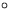and blaze angle of 80and with 166 grooves per mm. The grating is illuminated by a TE polarized wave of wavelength 155 nm under an incidence angle of 80.Using classical FEM, no acceptable result can be obtained with less than 2000000 unknowns.

Table 1: Results of GDPOTR for echelle grid
 degrees of freedom memory for solver reflected efficiency of order -74 105785 0.35 GB 37.931045 263624 0.70 GB 67.384460 559800 1.98 GB 68.390312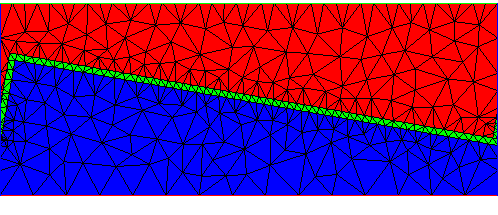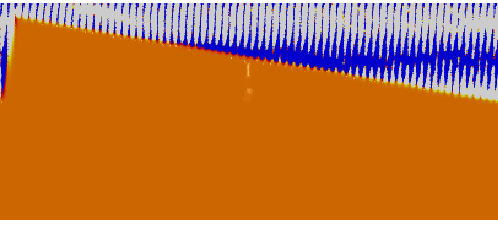#### 2. Modeling of 3D optical gratings

(G. Schmidt).

In  the diffraction by biperiodic structures is considered which consist of in general anisotropic materials, are periodic in two not necessarily orthogonal directions and separate two regions with constant dielectric coefficients. The scattering of plane waves is modeled by time-harmonic Maxwell equations with special quasi-periodic radiation conditions.

The biperiodic diffraction problem is to find, for a given incident wave of arbitrary polarization, the scattered far-field pattern, i.e. the diffraction efficiencies and the states of polarization of all propagating diffracted waves. This problem is of considerable interest in different areas of modern optics, where such doubly periodic structures are called crossed anisotropic gratings. The numerical modeling of those devices has recently received considerable attention within the engineering community.

The analysis is based on a variational formulation for the magnetic field in a bounded biperiodic cell with nonlocal boundary conditions which is equivalent to Maxwell's system. Since the variational problem is strongly elliptic, quite complete results can be obtained concerning the solvability and uniqueness of the biperiodic diffraction problem. It is shown that the biperiodic diffraction problem for general structures is always solvable and that the solution is unique with the possibly exception of a discrete set of wavelengths converging to infinity. If the structure contains absorbing materials then the problem is uniquely solvable for all wavelengths. Moreover, the strong ellipticity of the variational formulation justifies the use of finite element methods to solve the biperiodic diffraction problem.

#### 3. Reconstruction of grating profiles

(G. Bruckner, J. Elschner, G. Schmidt).

The problem of recovering a periodic structure from knowledge of the scattered field occurs in many applications, e.g., in diffractive optics (), radar imaging, and nondestructive testing. We studied the scattering of monochromatic plane waves by a perfectly reflecting diffraction grating in an isotropic lossless medium, which is modeled by the Dirichlet problem (transverse electric polarization) or the Neumann problem (transverse magnetic polarization) for the periodic Helmholtz equation.

Let the profile of the diffraction grating be given by the curve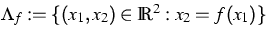where f is a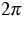-periodic Lipschitz function. Suppose that a plane wave given by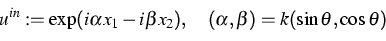is incident on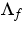from the top, where the wave number k is a positive constant and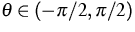is the incident angle. The inverse problem or the profile reconstruction problem can be formulated as follows.

(IP): Determine the profile function f from the knowledge of one wave number k, one incident direction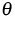and the total field u|x2=b on a straight line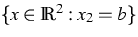with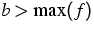.

Note that this problem also involves near-field measurements since the evanescent modes cannot be measured far away from the grating profile. The global uniqueness in problem (IP) is known if the wave number or the amplitude of the grating are sufficiently small (). In general, global uniqueness may not be true. This can be seen from the simple counterexample of the scattering of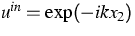when one moves the flat grating in certain multiples of the wavelength. Even if one excludes this flat case, the global uniqueness is not known with a single wave number.

We were able to solve this open problem in the practically important case of piecewise linear grating profiles . A preliminary result for rectangular diffractive structures (e.g., for binary gratings) was established in . The proof relies on the analyticity of solutions to the Helmholtz equation and the Rayleigh expansion of the scattered field.

Furthermore, in the case of the inverse Dirichlet problem, we continued to study the performance of reconstruction algorithms for grating profiles given by truncated Fourier series or piecewise linear profile functions. To this end, an unknown density function is computed from measured near-field data first, which allows us to represent the scattered field as a single layer potential. This is a severely ill-posed linear problem with a known singular value decomposition that helps to solve it at a low numerical expense. Then the computed density function is used as input to a nonlinear least squares problem which determines the unknown profile as the location of the zeros of the total field and is solved by the Gauss-Newton method. This approach, originally due to Kirsch and Kress in the case of acoustic obstacle scattering, avoids the solution of a direct problem in each iteration. Additionally, since only the grating parameters need be improved in each Gauss-Newton step, the linear system is of low dimension.

Numerical experiments with synthetic and noisy data have demonstrated the efficiency of the inversion algorithm . The case when the minimization of the Tikhonov functional for the linear problem and the defect minimization of the Dirichlet condition is combined into one cost functional has been treated in ; see also  for its mathematical foundation in the general case of Lipschitz grating profiles.

#### 4. Inverse medium problems

(J. Elschner, A. Rathsfeld).

A more challenging inverse problem is the reconstruction from far-field measurements of gratings with unknown refractive index, i.e. the refractive index is to be determined as a function of the points in the cross-section domain. Again, we have reduced this task to an optimization problem. Now the electric field is included in the set of arguments and the residual of the Helmholtz equation is added as a penalty term into the objective functional. For a fixed regularization parameter, we proved the solvability of the optimization problem, and, for the parameter tending to zero, we showed the convergence to a minimizer. Using FE discretization and conjugate gradient or SQP, numerical experiments confirmed these results (cf. ). Of course, due to the large number of local minima for the high-dimensional problem, a good initial solution is required. The left picture of Figure 3 shows the refractive index function of a lamellar grating with substrate of index 1.5, with a small rectangle of index 1.5, and with a higher rectangle of index 1.3. Above the grating we assume air with index 1 and the period is 1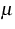m. For our reconstruction, we illuminated the grating by light with a wave length of 633 nm. The far-field data was chosen as the efficiencies and phase shifts corresponding to the reflected and transmitted modes for plane wave illumination incident from 25 different directions. The reconstructed function is shown in the right picture of Figure 3 and exhibits similar approximation properties like reconstructions in inverse scattering problems.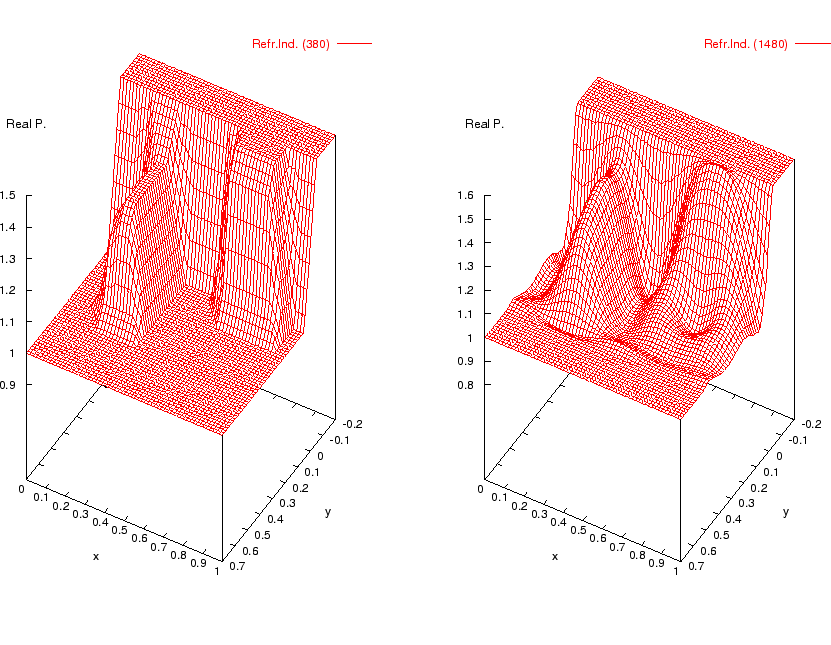References:

1. G. BRUCKNER, J. ELSCHNER, A two-step algorithm for the reconstruction of perfectly reflecting periodic profiles, WIAS Preprint no. 769, 2002, to appear in: Inverse Problems.
2. G. BRUCKNER, J. ELSCHNER, M. YAMAMOTO, An optimization method for grating profile reconstruction, to appear in: Proc. 3rd ISAAC Congress.
3. J. ELSCHNER, M. YAMAMOTO, An inverse problem in periodic diffractive optics: Reconstruction of Lipschitz grating profiles, Appl. Anal., 81 (2002), pp. 1307-1328.
4. J. ELSCHNER, R. HINDER, G. SCHMIDT, Finite element solution of conical diffraction problems, Adv. Comput. Math., 16 (2002), pp. 139-156.
5. J. ELSCHNER, G.C. HSIAO, R. RATHSFELD, Grating profile reconstruction based on finite elements and optimization techniques, WIAS Preprint no. 785, 2002.
6. J. ELSCHNER, G. SCHMIDT, M. YAMAMOTO, Global uniqueness in determining rectangular periodic structures by scattering data with a single wave number, WIAS Preprint no. 773, 2002.
7., An inverse problem in periodic diffractive optics: Global uniqueness with a single wave number, submitted.
8. F. HETTLICH, A. KIRSCH, Schiffer's theorem in inverse scattering for periodic structures, Inverse Problems, 13 (1997), pp. 351-361.
9. G. SCHMIDT, On the diffraction by biperiodic anisotropic structures, WIAS Preprint no. 751, 2002, to appear in: Appl. Anal.
10. J. TURUNEN, F. WYROWSKI (EDS.), Diffractive Optics for Industrial and Commercial Applications, Akademie-Verlag, Berlin, 1997.

 [Next]: Optimization problems with random and nonsmooth
 [Up]: Project descriptions
 [Previous]: Project descriptions
[Contents]   [Index]

LaTeX typesetting by I. Bremer
5/16/2003